# Spherically Symmetric Configurations (Part I)

If the self-gravitating configuration that we wish to construct is spherically symmetric, then the coupled set of multidimensional, partial differential equations that serve as our principal governing equations can be simplified to a coupled set of one-dimensional, ordinary differential equations. This is accomplished by expressing each of the multidimensional spatial operators — gradient, divergence, and Laplacian — in spherical coordinates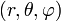$~(r, \theta, \varphi)$ then setting to zero all derivatives that are taken with respect to the angular coordinates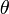$~\theta$ and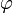$~\varphi$. After making this simplification, our governing equations become,

Equation of Continuity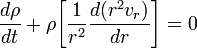$\frac{d\rho}{dt} + \rho \biggl[\frac{1}{r^2}\frac{d(r^2 v_r)}{dr} \biggr] = 0$

Euler Equation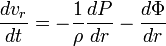$\frac{dv_r}{dt} = - \frac{1}{\rho}\frac{dP}{dr} - \frac{d\Phi}{dr}$

First Law of Thermodynamics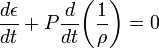$~\frac{d\epsilon}{dt} + P \frac{d}{dt} \biggl(\frac{1}{\rho}\biggr) = 0$

Poisson Equation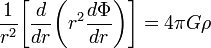$\frac{1}{r^2} \biggl[\frac{d }{dr} \biggl( r^2 \frac{d \Phi}{dr} \biggr) \biggr] = 4\pi G \rho$